# Data Visualization with Python

• Last Updated : 22 Jul, 2021

In today’s world, a lot of data is being generated on a daily basis. And sometimes to analyze this data for certain trends, patterns may become difficult if the data is in its raw format. To overcome this data visualization comes into play. Data visualization provides a good, organized pictorial representation of the data which makes it easier to understand, observe, analyze. In this tutorial, we will discuss how to visualize data using Python.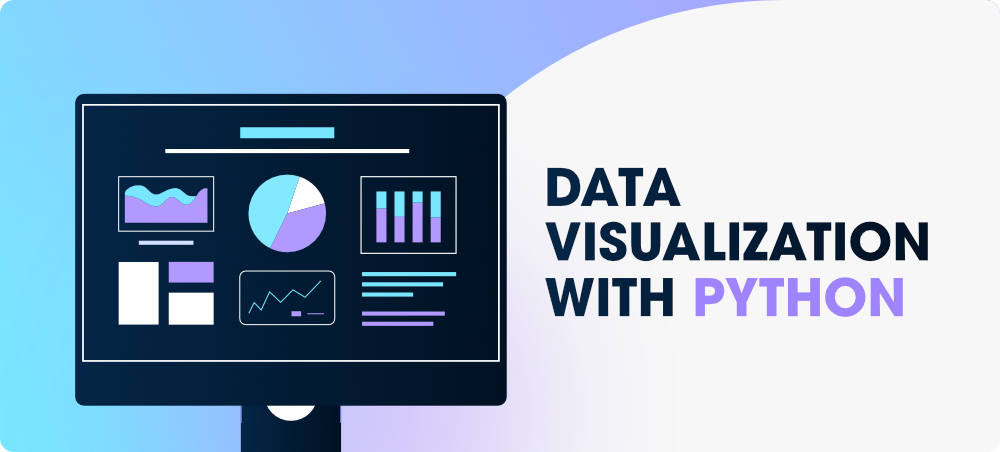Attention geek! Strengthen your foundations with the Python Programming Foundation Course and learn the basics.

To begin with, your interview preparations Enhance your Data Structures concepts with the Python DS Course. And to begin with your Machine Learning Journey, join the Machine Learning - Basic Level Course

Python provides various libraries that come with different features for visualizing data. All these libraries come with different features and can support various types of graphs. In this tutorial, we will be discussing four such libraries.

• Matplotlib
• Seaborn
• Bokeh
• Plotly

We will discuss these libraries one by one and will plot some most commonly used graphs.

Note: If you want to learn in-depth information about these libraries you can follow their complete tutorial.

Before diving into these libraries, at first, we will need a database to plot the data. We will be using the tips database for this complete tutorial. Let’s discuss see a brief about this database.

## Database Used

### Tips Database

Tips database is the record of the tip given by the customers in a restaurant for two and a half months in the early 1990s. It contains 6 columns such as total_bill, tip, sex, smoker, day, time, size.

Example:

## Python3

 `import` `pandas as pd`` ` ` ` `# reading the database``data ``=` `pd.read_csv(``"tips.csv"``)`` ` `# printing the top 10 rows``display(data.head(``10``))`

Output: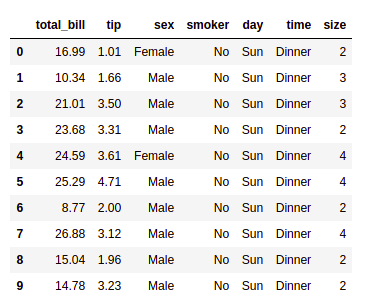## Matplotlib

Matplotlib is an easy-to-use, low-level data visualization library that is built on NumPy arrays. It consists of various plots like scatter plot, line plot, histogram, etc. Matplotlib provides a lot of flexibility.

To install this type the below command in the terminal.

`pip install matplotlib`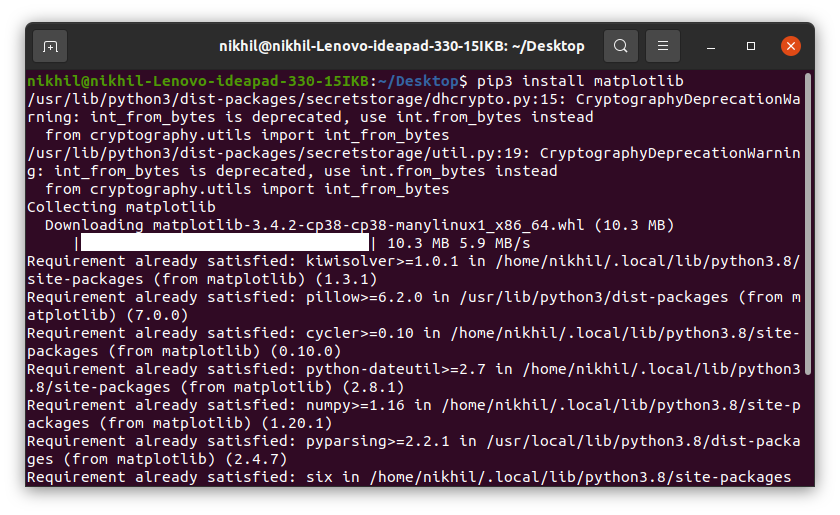Refer to the below articles to get more information setting up an environment with Matplotlib.

After installing Matplotlib, let’s see the most commonly used plots using this library.

### Scatter Plot

Scatter plots are used to observe relationships between variables and uses dots to represent the relationship between them. The scatter() method in the matplotlib library is used to draw a scatter plot.

Example:

## Python3

 `import` `pandas as pd``import` `matplotlib.pyplot as plt`` ` ` ` `# reading the database``data ``=` `pd.read_csv(``"tips.csv"``)`` ` `# Scatter plot with day against tip``plt.scatter(data[``'day'``], data[``'tip'``])`` ` `# Adding Title to the Plot``plt.title(``"Scatter Plot"``)`` ` `# Setting the X and Y labels``plt.xlabel(``'Day'``)``plt.ylabel(``'Tip'``)`` ` `plt.show()`

Output: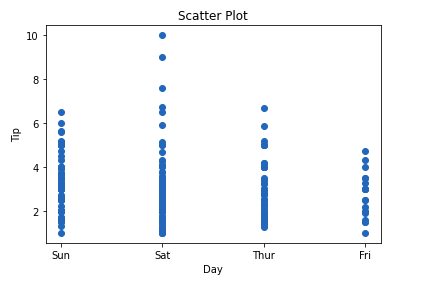This graph can be more meaningful if we can add colors and also change the size of the points. We can do this by using the c and s parameter respectively of the scatter function. We can also show the color bar using the colorbar() method.

Example:

## Python3

 `import` `pandas as pd``import` `matplotlib.pyplot as plt`` ` ` ` `# reading the database``data ``=` `pd.read_csv(``"tips.csv"``)`` ` `# Scatter plot with day against tip``plt.scatter(data[``'day'``], data[``'tip'``], c``=``data[``'size'``], ``            ``s``=``data[``'total_bill'``])`` ` `# Adding Title to the Plot``plt.title(``"Scatter Plot"``)`` ` `# Setting the X and Y labels``plt.xlabel(``'Day'``)``plt.ylabel(``'Tip'``)`` ` `plt.colorbar()`` ` `plt.show()`

Output: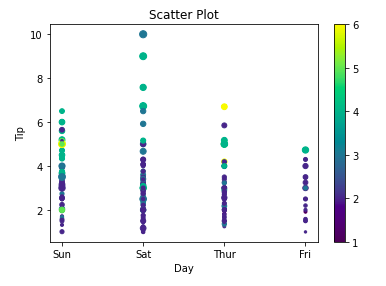### Line Chart

Line Chart is used to represent a relationship between two data X and Y on a different axis. It is plotted using the plot() function. Let’s see the below example.

Example:

## Python3

 `import` `pandas as pd``import` `matplotlib.pyplot as plt`` ` ` ` `# reading the database``data ``=` `pd.read_csv(``"tips.csv"``)`` ` `# Scatter plot with day against tip``plt.plot(data[``'tip'``])``plt.plot(data[``'size'``])`` ` `# Adding Title to the Plot``plt.title(``"Scatter Plot"``)`` ` `# Setting the X and Y labels``plt.xlabel(``'Day'``)``plt.ylabel(``'Tip'``)`` ` `plt.show()`

Output: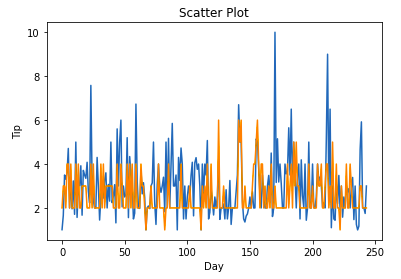### Bar Chart

A bar plot or bar chart is a graph that represents the category of data with rectangular bars with lengths and heights that is proportional to the values which they represent. It can be created using the bar() method.

Example:

## Python3

 `import` `pandas as pd``import` `matplotlib.pyplot as plt`` ` ` ` `# reading the database``data ``=` `pd.read_csv(``"tips.csv"``)`` ` `# Bar chart with day against tip``plt.bar(data[``'day'``], data[``'tip'``])`` ` `plt.title(``"Bar Chart"``)`` ` `# Setting the X and Y labels``plt.xlabel(``'Day'``)``plt.ylabel(``'Tip'``)`` ` `# Adding the legends``plt.show()`

Output: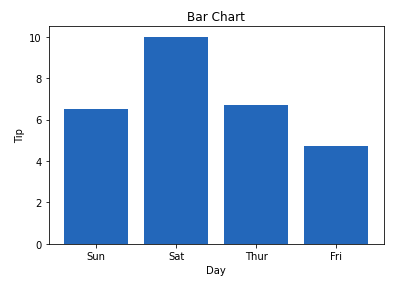### Histogram

A histogram is basically used to represent data in the form of some groups. It is a type of bar plot where the X-axis represents the bin ranges while the Y-axis gives information about frequency. The hist() function is used to compute and create a histogram. In histogram, if we pass categorical data then it will automatically compute the frequency of that data i.e. how often each value occurred.

Example:

## Python3

 `import` `pandas as pd``import` `matplotlib.pyplot as plt`` ` ` ` `# reading the database``data ``=` `pd.read_csv(``"tips.csv"``)`` ` `# hostogram of total_bills``plt.hist(data[``'total_bill'``])`` ` `plt.title(``"Histogram"``)`` ` `# Adding the legends``plt.show()`

Output: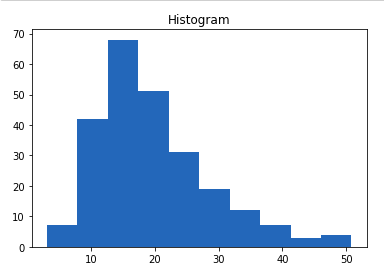Note: For complete Matplotlib Tutorial, refer Matplotlib Tutorial

## Seaborn

Seaborn is a high-level interface built on top of the Matplotlib. It provides beautiful design styles and color palettes to make more attractive graphs.

To install seaborn type the below command in the terminal.

`pip install seaborn`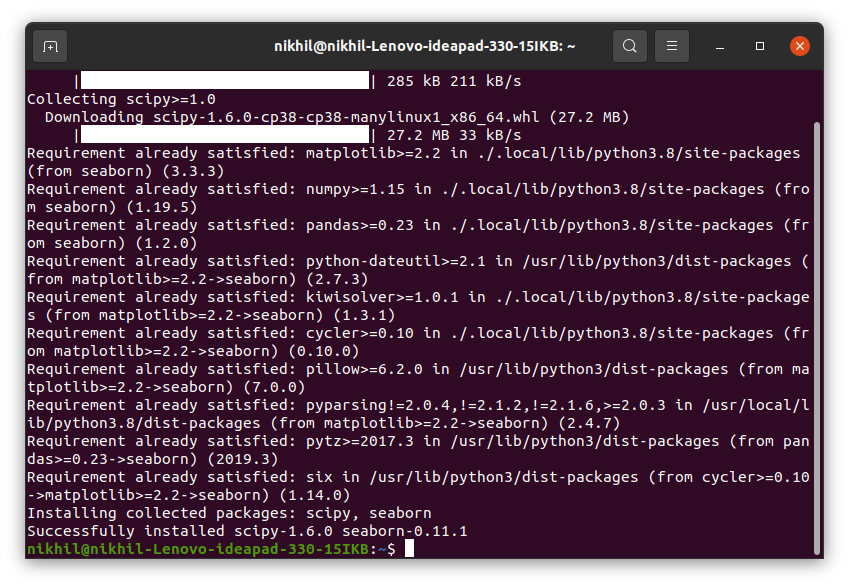Seaborn is built on the top of Matplotlib, therefore it can be used with the Matplotlib as well. Using both Matplotlib and Seaborn together is a very simple process. We just have to invoke the Seaborn Plotting function as normal, and then we can use Matplotlib’s customization function.

Note: Seaborn comes loaded with dataset such as tips, iris, etc. but for the sake of this tutorial we will use Pandas for loading these datasets.

Example:

## Python3

 `# importing packages``import` `seaborn as sns``import` `matplotlib.pyplot as plt``import` `pandas as pd`` ` ` ` `# reading the database``data ``=` `pd.read_csv(``"tips.csv"``)`` ` `# draw lineplot``sns.lineplot(x``=``"sex"``, y``=``"total_bill"``, data``=``data)`` ` `# setting the title using Matplotlib``plt.title(``'Title using Matplotlib Function'``)`` ` `plt.show()`

Output: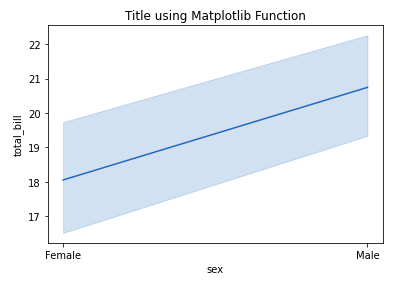### Scatter Plot

Scatter plot is plotted using the scatterplot() method. This is similar to Matplotlib, but additional argument data is required.

Example:

## Python3

 `# importing packages``import` `seaborn as sns``import` `matplotlib.pyplot as plt``import` `pandas as pd`` ` `# reading the database``data ``=` `pd.read_csv(``"tips.csv"``)`` ` `sns.scatterplot(x``=``'day'``, y``=``'tip'``, data``=``data,)``plt.show()`

Output: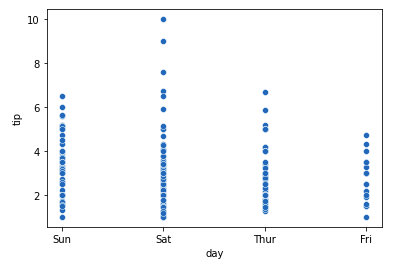You will find that while using Matplotlib it will a lot difficult if you want to color each point of this plot according to the sex. But in scatter plot it can be done with the help of hue argument.

Example:

## Python3

 `# importing packages``import` `seaborn as sns``import` `matplotlib.pyplot as plt``import` `pandas as pd`` ` `# reading the database``data ``=` `pd.read_csv(``"tips.csv"``)`` ` `sns.scatterplot(x``=``'day'``, y``=``'tip'``, data``=``data,``               ``hue``=``'sex'``)``plt.show()`

Output: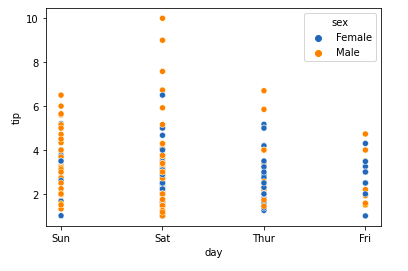### Line Plot

Line Plot in Seaborn plotted using the lineplot() method.  In this, we can pass only the data argument also.

Example:

## Python3

 `# importing packages``import` `seaborn as sns``import` `matplotlib.pyplot as plt``import` `pandas as pd`` ` ` ` `# reading the database``data ``=` `pd.read_csv(``"tips.csv"``)`` ` `sns.lineplot(x``=``'day'``, y``=``'tip'``, data``=``data)``plt.show()`

Output: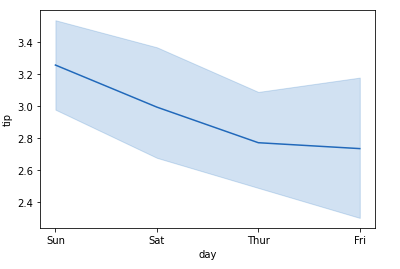Example 2:

## Python3

 `# importing packages``import` `seaborn as sns``import` `matplotlib.pyplot as plt``import` `pandas as pd`` ` ` ` `# reading the database``data ``=` `pd.read_csv(``"tips.csv"``)`` ` `# using only data attribute``sns.lineplot(data``=``data.drop([``'total_bill'``], axis``=``1``))``plt.show()`

Output: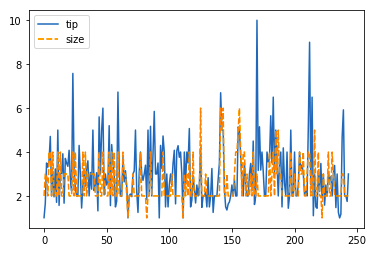### Bar Plot

Bar Plot in Seaborn can be created using the barplot() method.

Example:

## Python3

 `# importing packages``import` `seaborn as sns``import` `matplotlib.pyplot as plt``import` `pandas as pd`` ` ` ` `# reading the database``data ``=` `pd.read_csv(``"tips.csv"``)`` ` `sns.barplot(x``=``'day'``,y``=``'tip'``, data``=``data, ``            ``hue``=``'sex'``)`` ` `plt.show()`

Output: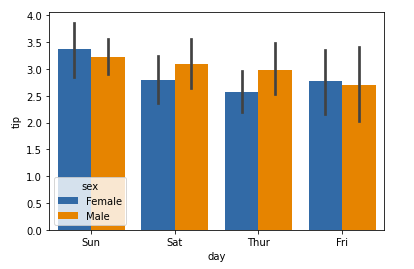### Histogram

The histogram in Seaborn can be plotted using the histplot() function.

Example:

## Python3

 `# importing packages``import` `seaborn as sns``import` `matplotlib.pyplot as plt``import` `pandas as pd`` ` ` ` `# reading the database``data ``=` `pd.read_csv(``"tips.csv"``)`` ` `sns.histplot(x``=``'total_bill'``, data``=``data, kde``=``True``, hue``=``'sex'``)`` ` `plt.show()`

Output: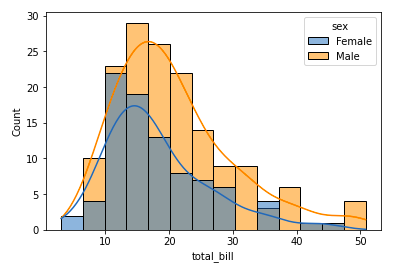After going through all these plots you must have noticed that customizing plots using Seaborn is a lot more easier than using Matplotlib. And it is also built over matplotlib then we can also use matplotlib functions while using Seaborn.

Note: For complete Seaborn Tutorial, refer Python Seaborn Tutorial

## Bokeh

Let’s move on to the third library of our list. Bokeh is mainly famous for its interactive charts visualization. Bokeh renders its plots using HTML and JavaScript that uses modern web browsers for presenting elegant, concise construction of novel graphics with high-level interactivity.

To install this type the below command in the terminal.

`pip install bokeh`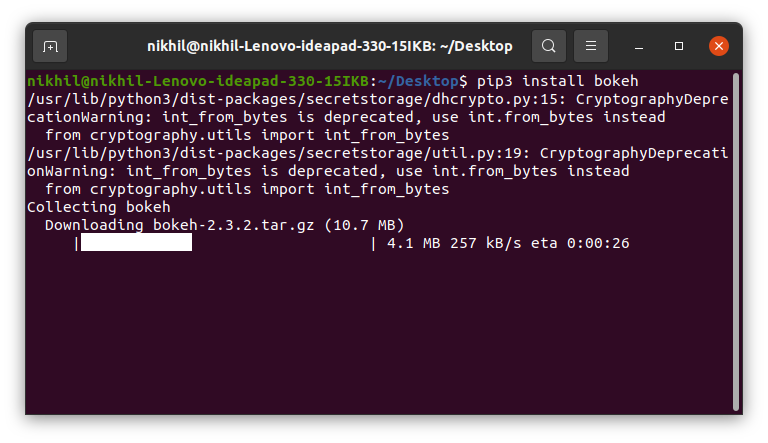### Scatter Plot

Scatter Plot in Bokeh can be plotted using the scatter() method of the plotting module. Here pass the x and y coordinates respectively.

Example:

## Python3

 `# importing the modules``from` `bokeh.plotting ``import` `figure, output_file, show``from` `bokeh.palettes ``import` `magma``import` `pandas as pd`` ` ` ` `# instantiating the figure object``graph ``=` `figure(title ``=` `"Bokeh Scatter Graph"``)`` ` `# reading the database``data ``=` `pd.read_csv(``"tips.csv"``)`` ` `color ``=` `magma(``256``)`` ` `# plotting the graph``graph.scatter(data[``'total_bill'``], data[``'tip'``], color``=``color)`` ` `# displaying the model``show(graph)`

Output: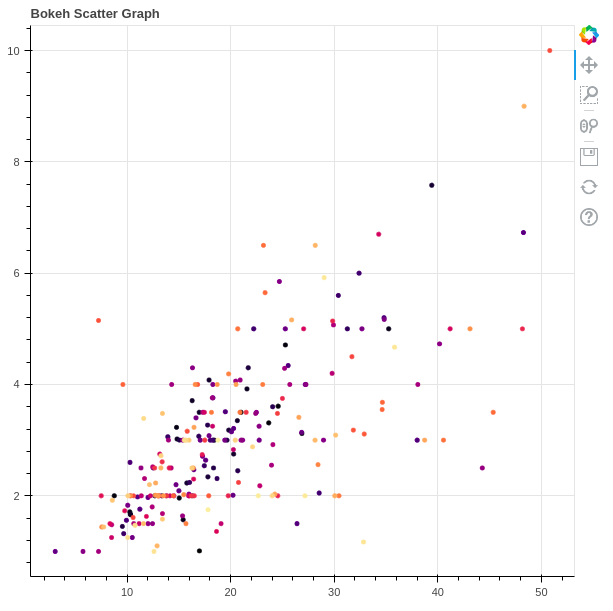### Line Chart

A line plot can be created using the line() method of the plotting module.

Example:

## Python3

 `# importing the modules``from` `bokeh.plotting ``import` `figure, output_file, show``import` `pandas as pd`` ` ` ` `# instantiating the figure object``graph ``=` `figure(title ``=` `"Bokeh Bar Chart"``)`` ` `# reading the database``data ``=` `pd.read_csv(``"tips.csv"``)`` ` `# Count of each unique value of``# tip column``df ``=` `data[``'tip'``].value_counts()`` ` `# plotting the graph``graph.line(df, data[``'tip'``])`` ` `# displaying the model``show(graph)`

Output: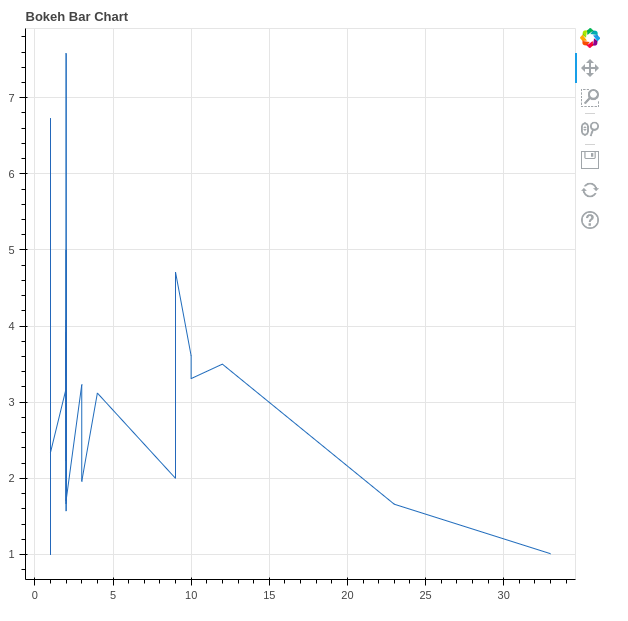### Bar Chart

Bar Chart can be of two types horizontal bars and vertical bars. Each can be created using the hbar() and vbar() functions of the plotting interface respectively.

Example:

## Python3

 `# importing the modules``from` `bokeh.plotting ``import` `figure, output_file, show``import` `pandas as pd`` ` ` ` `# instantiating the figure object``graph ``=` `figure(title ``=` `"Bokeh Bar Chart"``)`` ` `# reading the database``data ``=` `pd.read_csv(``"tips.csv"``)`` ` `# plotting the graph``graph.vbar(data[``'total_bill'``], top``=``data[``'tip'``])`` ` `# displaying the model``show(graph)`

Output: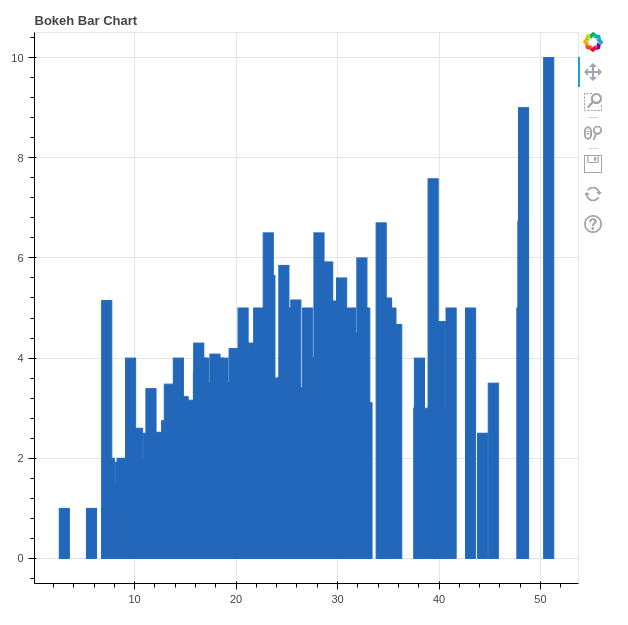### Interactive Data Visualization

One of the key features of Bokeh is to add interaction to the plots. Let’s see various interactions that can be added.

#### Interactive Legends

click_policy property makes the legend interactive. There are two types of interactivity –

• Hiding: Hides the Glyphs.
• Muting: Hiding the glyph makes it vanish completely, on the other hand, muting the glyph just de-emphasizes the glyph based on the parameters.

Example:

## Python3

 `# importing the modules``from` `bokeh.plotting ``import` `figure, output_file, show``import` `pandas as pd`` ` ` ` `# instantiating the figure object``graph ``=` `figure(title ``=` `"Bokeh Bar Chart"``)`` ` `# reading the database``data ``=` `pd.read_csv(``"tips.csv"``)`` ` `# plotting the graph``graph.vbar(data[``'total_bill'``], top``=``data[``'tip'``], ``           ``legend_label ``=` `"Bill VS Tips"``, color``=``'green'``)`` ` `graph.vbar(data[``'tip'``], top``=``data[``'size'``], ``           ``legend_label ``=` `"Tips VS Size"``, color``=``'red'``)`` ` `graph.legend.click_policy ``=` `"hide"`` ` `# displaying the model``show(graph)`

Output: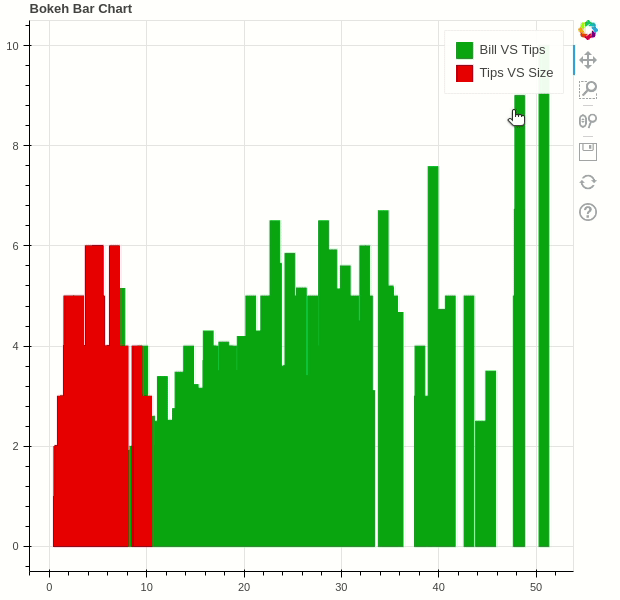Bokeh provides GUI features similar to HTML forms like buttons, sliders, checkboxes, etc. These provide an interactive interface to the plot that allows changing the parameters of the plot, modifying plot data, etc. Let’s see how to use and add some commonly used widgets.

• Buttons: This widget adds a simple button widget to the plot. We have to pass a custom JavaScript function to the CustomJS() method of the models class.
• CheckboxGroup: Adds a standard check box to the plot. Similarly to buttons we have to pass the custom JavaScript function to the CustomJS() method of the models class.

Example:

## Python3

 `from` `bokeh.io ``import` `show``from` `bokeh.models ``import` `Button, CheckboxGroup, RadioGroup, CustomJS`` ` `button ``=` `Button(label``=``"GFG"``)`` ` `button.js_on_click(CustomJS(``    ``code``=``"console.log('button: click!', this.toString())"``))`` ` `# Labels for checkbox and radio``# buttons``L ``=` `[``"First"``, ``"Second"``, ``"Third"``]`` ` `# the active parameter sets checks the selected value``# by default``checkbox_group ``=` `CheckboxGroup(labels``=``L, active``=``[``0``, ``2``])`` ` `checkbox_group.js_on_click(CustomJS(code``=``"""``    ``console.log('checkbox_group: active=' + this.active, this.toString())``"""``))`` ` `# the active parameter sets checks the selected value``# by default``radio_group ``=` `RadioGroup(labels``=``L, active``=``1``)`` ` `radio_group.js_on_click(CustomJS(code``=``"""``    ``console.log('radio_group: active=' + this.active, this.toString())``"""``))`` ` `show(button)``show(checkbox_group)``show(radio_group)`

Output: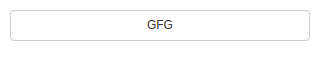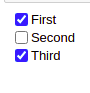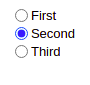Note: All these buttons will be opened on a new tab.

• Sliders: Adds a slider to the plot. It also needs a custom JavaScript function.

Example:

## Python3

 `from` `bokeh.io ``import` `show``from` `bokeh.models ``import` `CustomJS, Slider`` ` `slider ``=` `Slider(start``=``1``, end``=``20``, value``=``1``, step``=``2``, title``=``"Slider"``)`` ` `slider.js_on_change(``"value"``, CustomJS(code``=``"""``    ``console.log('slider: value=' + this.value, this.toString())``"""``))`` ` `show(slider)`

Output: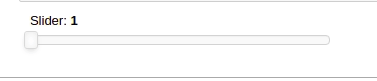Similarly, much more widgets are available like a dropdown menu or tabs widgets can be added.

Note: For complete Bokeh tutorial, refer Python Bokeh tutorial – Interactive Data Visualization with Bokeh

## Plotly

This is the last library of our list and you might be wondering why plotly. Here’s why –

• Potly has hover tool capabilities that allow us to detect any outliers or anomalies in numerous data points.
• It allows more customization.
• It makes the graph visually more attractive.

To install it type the below command in the terminal.

`pip install plotly`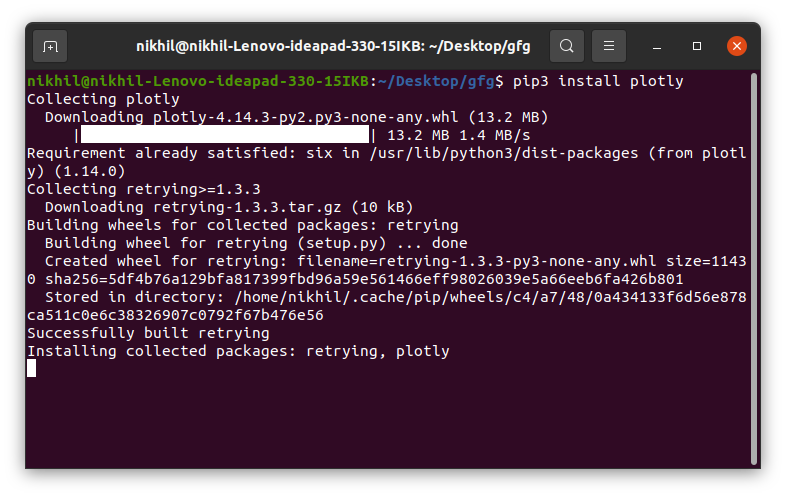### Scatter Plot

Scatter plot in Plotly can be created using the scatter() method of plotly.express. Like Seaborn, an extra data argument is also required here.

Example:

## Python3

 `import` `plotly.express as px``import` `pandas as pd`` ` `# reading the database``data ``=` `pd.read_csv(``"tips.csv"``)`` ` `# plotting the scatter chart``fig ``=` `px.scatter(data, x``=``"day"``, y``=``"tip"``, color``=``'sex'``)`` ` `# showing the plot``fig.show()`

Output: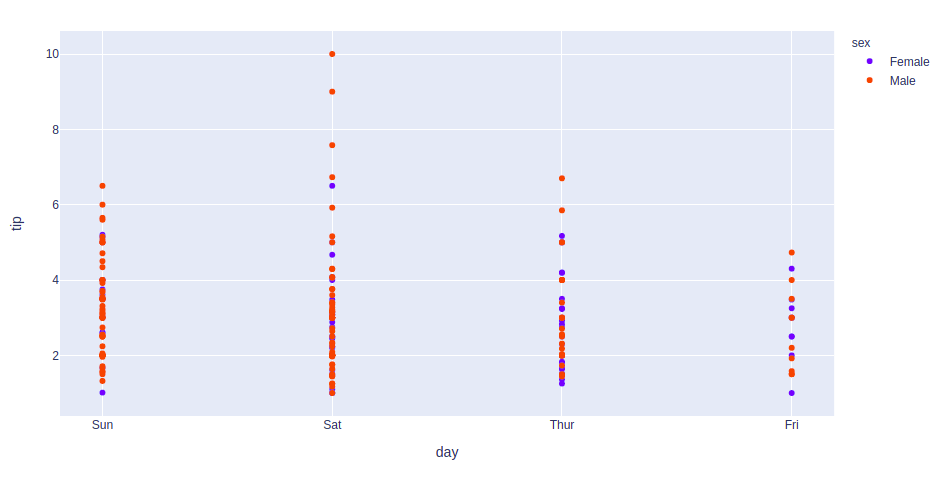### Line Chart

Line plot in Plotly is much accessible and illustrious annexation to plotly which manage a variety of types of data and assemble easy-to-style statistic. With px.line each data position is represented as a vertex

Example:

## Python3

 `import` `plotly.express as px``import` `pandas as pd`` ` `# reading the database``data ``=` `pd.read_csv(``"tips.csv"``)`` ` `# plotting the scatter chart``fig ``=` `px.line(data, y``=``'tip'``, color``=``'sex'``)`` ` `# showing the plot``fig.show()`

Output: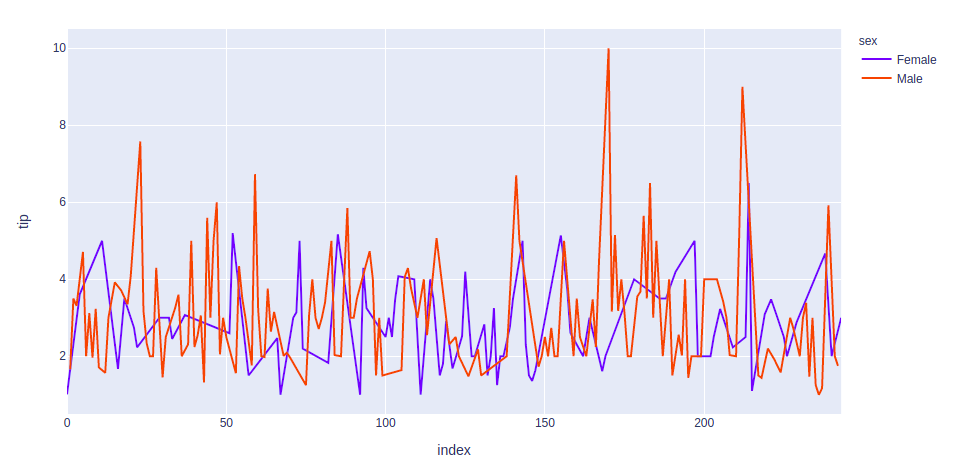### Bar Chart

Bar Chart in Plotly can be created using the bar() method of plotly.express class.

Example:

## Python3

 `import` `plotly.express as px``import` `pandas as pd`` ` `# reading the database``data ``=` `pd.read_csv(``"tips.csv"``)`` ` `# plotting the scatter chart``fig ``=` `px.bar(data, x``=``'day'``, y``=``'tip'``, color``=``'sex'``)`` ` `# showing the plot``fig.show()`

Output: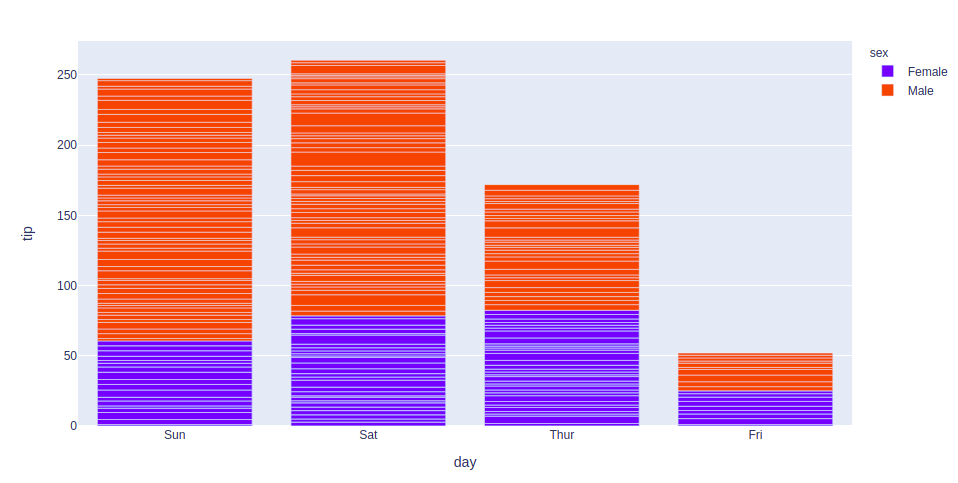### Histogram

In plotly, histograms can be created using the histogram() function of the plotly.express class.

Example:

## Python3

 `import` `plotly.express as px``import` `pandas as pd`` ` `# reading the database``data ``=` `pd.read_csv(``"tips.csv"``)`` ` `# plotting the scatter chart``fig ``=` `px.histogram(data, x``=``'total_bill'``, color``=``'sex'``)`` ` `# showing the plot``fig.show()`

Output: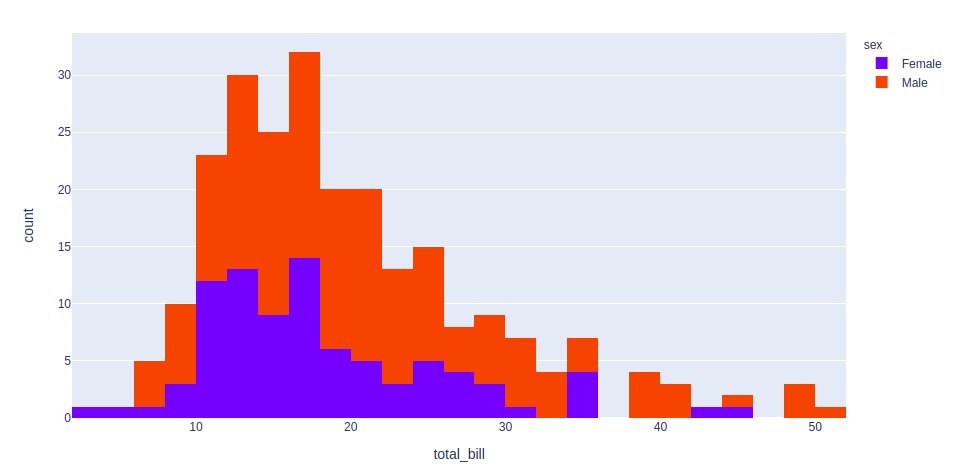Just like Bokeh, plotly also provides various interactions. Let’s discuss a few of them.

Creating Dropdown Menu: A drop-down menu is a part of the menu-button which is displayed on a screen all the time. Every menu button is associated with a Menu widget that can display the choices for that menu button when clicked on it. In plotly, there are 4 possible methods to modify the charts by using updatemenu method.

• restyle: modify data or data attributes
• relayout: modify layout attributes
• update: modify data and layout attributes
• animate: start or pause an animation

Example:

## Python3

 `import` `plotly.graph_objects as px``import` `pandas as pd`` ` `# reading the database``data ``=` `pd.read_csv(``"tips.csv"``)`` ` ` ` `plot ``=` `px.Figure(data``=``[px.Scatter(``    ``x``=``data[``'day'``],``    ``y``=``data[``'tip'``],``    ``mode``=``'markers'``,)``])`` ` `# Add dropdown``plot.update_layout(``    ``updatemenus``=``[``        ``dict``(``            ``buttons``=``list``([``                ``dict``(``                    ``args``=``[``"type"``, ``"scatter"``],``                    ``label``=``"Scatter Plot"``,``                    ``method``=``"restyle"``                ``),``                ``dict``(``                    ``args``=``[``"type"``, ``"bar"``],``                    ``label``=``"Bar Chart"``,``                    ``method``=``"restyle"``                ``)``            ``]),``            ``direction``=``"down"``,``        ``),``    ``]``)`` ` `plot.show()`

Output: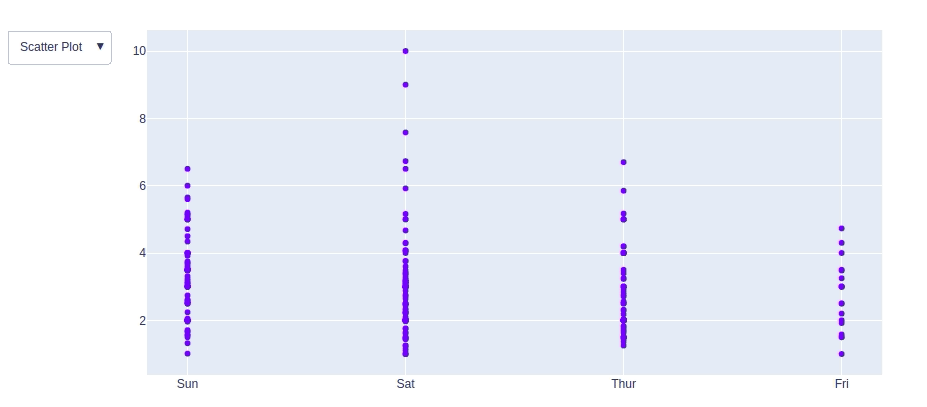Adding Buttons: In plotly, actions custom Buttons are used to quickly make actions directly from a record. Custom Buttons can be added to page layouts in CRM, Marketing, and Custom Apps. There are also 4 possible methods that can be applied in custom buttons:

• restyle: modify data or data attributes
• relayout: modify layout attributes
• update: modify data and layout attributes
• animate: start or pause an animation

Example:

## Python3

 `import` `plotly.graph_objects as px``import` `pandas as pd`` ` `# reading the database``data ``=` `pd.read_csv(``"tips.csv"``)`` ` ` ` `plot ``=` `px.Figure(data``=``[px.Scatter(``    ``x``=``data[``'day'``],``    ``y``=``data[``'tip'``],``    ``mode``=``'markers'``,)``])`` ` `# Add dropdown``plot.update_layout(``    ``updatemenus``=``[``        ``dict``(``            ``type``=``"buttons"``,``            ``direction``=``"left"``,``            ``buttons``=``list``([``                ``dict``(``                    ``args``=``[``"type"``, ``"scatter"``],``                    ``label``=``"Scatter Plot"``,``                    ``method``=``"restyle"``                ``),``                ``dict``(``                    ``args``=``[``"type"``, ``"bar"``],``                    ``label``=``"Bar Chart"``,``                    ``method``=``"restyle"``                ``)``            ``]),``        ``),``    ``]``)`` ` `plot.show()`

Output: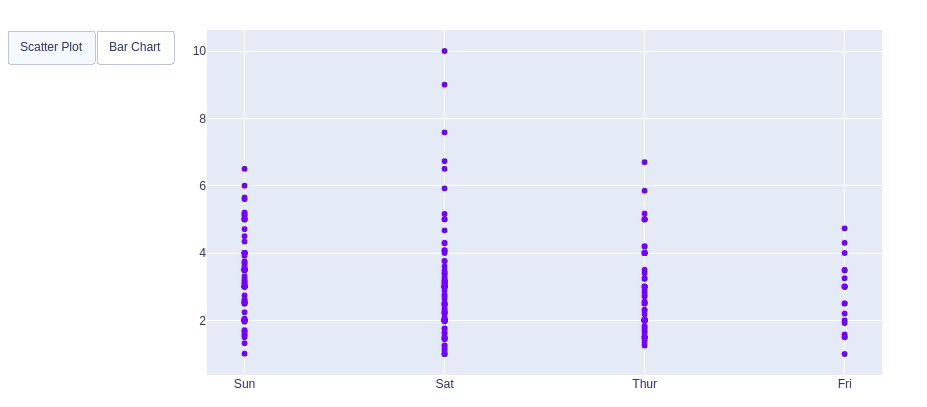Creating Sliders and Selectors:

In plotly, the range slider is a custom range-type input control. It allows selecting a value or a range of values between a specified minimum and maximum range. And the range selector is a tool for selecting ranges to display within the chart. It provides buttons to select pre-configured ranges in the chart. It also provides input boxes where the minimum and maximum dates can be manually input

Example:

## Python3

 `import` `plotly.graph_objects as px``import` `pandas as pd`` ` `# reading the database``data ``=` `pd.read_csv(``"tips.csv"``)`` ` `plot ``=` `px.Figure(data``=``[px.Scatter(``    ``y``=``data[``'tip'``],``    ``mode``=``'lines'``,)``])`` ` `plot.update_layout(``    ``xaxis``=``dict``(``        ``rangeselector``=``dict``(``            ``buttons``=``list``([``                ``dict``(count``=``1``,``                    ``step``=``"day"``,``                    ``stepmode``=``"backward"``),``            ``])``        ``),``        ``rangeslider``=``dict``(``            ``visible``=``True``        ``),``    ``)``)`` ` `plot.show()`

Output: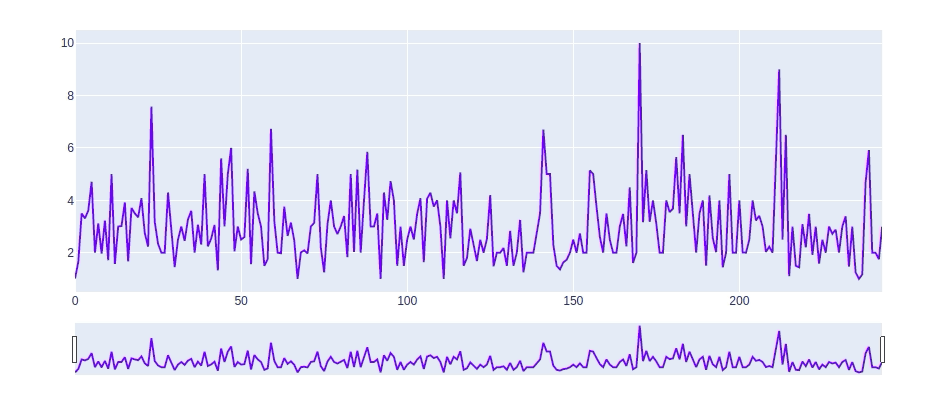Note: For complete Plotly tutorial, refer Python Plotly tutorial

## Conclusion

In this tutorial, we have plotted the tips dataset with the help of the four different plotting modules of Python namely Matplotlib, Seaborn, Bokeh, and Plotly. Each module showed the plot in its own unique way and each one has its own set of features like Matplotlib provides more flexibility but at the cost of writing more code whereas Seaborn being a high-level language provides allows one to achieve the same goal with a small amount of code. Each module can be used depending on the task we want to do.

My Personal Notes arrow_drop_up